#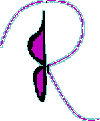Ellis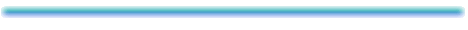Last Updated: 02 Apr 97JR Ellis, October 1992
Department of Mathematics
University of Utah

A thesis submitted to the faculty in partial fulfillment of the requirements for the degree of Master of Science

# ABSTRACT

An original four point compass and straight-edge construction is proposed for finding the square root of a line segment. The construction is geometrically derived and illustrated as changing a rectangle into a square in two stages. First, by turning the rectangle into the difference of two squares; and second, by subtracting them.

This idea is applied to forming a complete ordered field with the elements of the set of squares. After showing constructions for combining the squares, pictures which show the group properties follow. Finally, limit theory for an infinite sequence is shown graphically as a rectangular prism is iteratively changed into a cube.

The intent of this paper is to suggest that teaching math in elementary schools can be more conceptual and creative. By using approaches, as outlined here, education can be more effective and stimulating to young developing minds.

# ACKNOWLEDGMENT

I became exited when I discovered the square root construction that will be shown in this paper. The concept came naturally as I thought about the geometric interpretation of simple algebraic equations. I was most amazed that I hadn't thought of it sooner. After spending a lifetime in formal education classes, I hadn't really understood the simple concepts of algebra and geometry that men have known for thousands of years.

As I played with these ideas, I developed a simple system to see the properties of the real number system by using squares in order to visualize these properties. This thesis includes a video, which animates the square root construction and proof, and demonstrates the advantages and potential of computer graphics in education. The video was produced in the Art and Film department, with the help, cooperation and resources of the Mathematics and Education departments.

It is hoped that this idea will inspire a healthier approach to education of people learning mathematics. High school students are routinely taught how to factor the difference of two squares. However, the geometric interpretation of this equation is completely ignored. When I realized that the square of the hypotenuse of a right triangle equals the sum of the square of its legs really referred to four-sided squares, the square root construction followed easily. I was excited with the awareness. I was also sad that I had plodded through algebra without being exposed to its roots in geometry. I hope the pictures in this presentation will bring the equations to life.

I would like to thank Professor Robert E. Barnhill from the University of Utah for allowing me to pursue this path as a thesis and a film; and Professor Bevan K. Youse from Emory University, for introducing me to group theory and analysis and other recent private communications. And also, I would like to thank Dr. Andrew Mitz from NIH for giving me suggestions which helped me to write this thesis.

# INTRODUCTION

This thesis offers an original basic four point paper construction for square root by turning a rectangle into a square. The process first transforms the rectangle into the difference of two squares, so constructions and proofs for addition and subtraction of squares are presented first. The complete construction is given, including conceptualization and the proof. Also two compass-only procedures, which can be used in three of the four steps of the square root construction, are supplied so that the whole construction may be done with paper and compass and without needing a straight-edge. Even a construction to exact the measure of lines and squares is detailed, in order to exact real numbers from the root square.

A complete ordered field is formed by using squares as the elements of the set, with the operations of addition and multiplication. Constructions for these operations are shown, as well as division and subtraction. And there are pictures for properties like inverse, distributive, and multiplicative associativity.

Finally, the square root construction is extended by applying the procedure to an original iterative cube root construction. The limit theory for this infinite sequence uses nothing more advanced than an equation for the sum of a geometric progression. As with many of the geometric assertions in this paper, the algebraic proof is also included

The paper starts with an arithmetic application to the geometric interpretation of the difference to two squares. This shortcut can be used in normal arithmetic calculations.

# ARITHMETIC

Let's consider the arithmetic problem y multiplied by x. Sometimes it is easier to change the problem into
a**2 - b**2, where a = 1/2(x + y) and b = 1/2(x - y).
Let a represent the average of x and y, while b represents the deviation of x or y from this average, or x - a. This is useful when the squares are easy to compute. For example:

17 * 23 = (20 - 3) * (20 + 3) = 20**2 - 3**2 = 400 - 9 = 391

If the squares from one to one hundred are already memorized then two digit multiplication will be much faster. There is another advantage because there are fewer numbers to keep track of than with regular multiplication. The square may be computed with an arithmetic application of the binomial theorem for
n = 2, (a + b)**2 = a**2 + 2ab + b**2. (sorry... picture missing )

17**2 = (20 - 3)**2 = 20**2 + 2(-3)(20) + (-3)**2 = 400 - 120 + 9 = 289

The advantages of this technique maybe illustrated with another example. Here the challenge is to solve the following multiplication problem without using paper and pen:

24 * 26 = (25-1)(25+ 1) = 25**2 -1**2

Now we may forget 24 and 26 and remember 25 and 1. Next 25**2 = 625. And Finally, 625- 1 = 624. At no time were there more than six digits to keep track of at one time. First 24 and 26. Then 24, 25, 26. Then 24 and 25. Then 25 and 1. Lastly 625 and 1 before the solution of 624.

The standard way to multiply 24 and 26 is to first multiply 26 * 4 = 104 and then 26 * 20 = 520. And in order to add (104 + 520), there are more numbers to remember at one time than the previous proposed shortcut.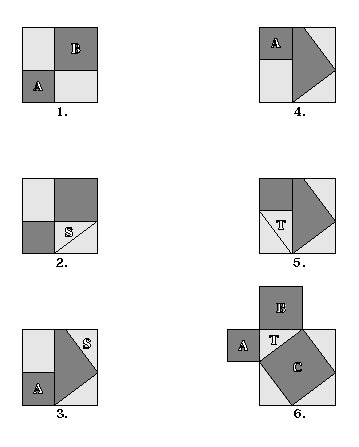A + B = C. To add squares A and B, construct a right triangle T as shown in figure 6. The hypotenuse square C is equal to the sum of the leg squares A and B. These figures illustrate this. The six large figure squares are all the same size. The two light colored rectangles from figure 1 are equal in area to the four light colored triangles in figure 6, because the triangles are one half the rectangles. Therefore what remains must also be equal. Namely, the sum of A and B from figure 1, and C from figure 6. Construct the right triangle T by drawing perpendicular lines, marking off the distances for the sides of A and B, then draw the side of C.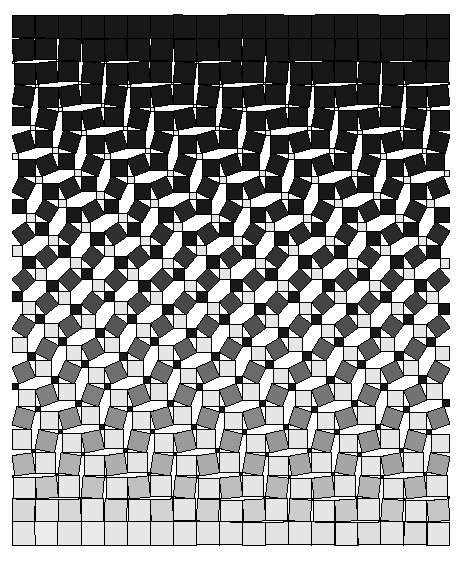# TRIGNOMETRIC DEFINITIONS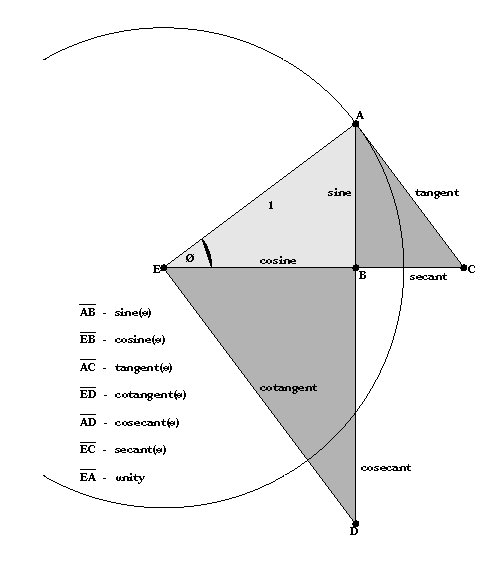The unity circle has center at point E and radius equal 1. Point A lies on the circumference of the circle. Drop a perpendicular line from A to the horizontal to label point B. Angle AEB equals angle theta. Then define the trigonometric functions below as the lengths of the line segments, and the inverse trig functions from those lengths. For example:
theta = arcsin AB

# SQUARE SUBTRACTION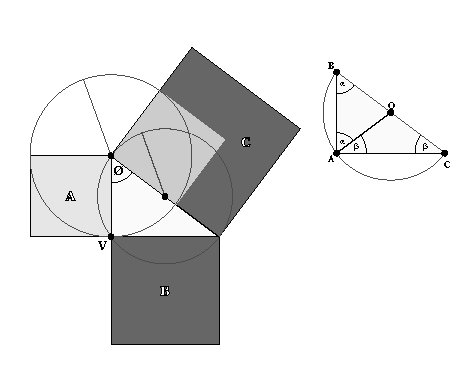Construct the difference square B by subtracting A from C. Form the right triangle by drawing a circle having as a diameter: a side of square C. Each point on this circle will form a right triangle with this side. Form square B by finding V as the intersection of the first circle, with the circle having its center at the vertex point of square C, and radius equal to the side of square A.
• Let a, b, and c represent the sides of the squares. This construction may be represented by the trigonometric definitions in an algebraic equation.
• Since cos theta = (a/c), then theta = arccos (a/c)
• And since sin theta= (b/c), then b = c * arcsin theta
• Substituting for theta , b = c * sin [arccos (a/c)]
• So c**2 - a**2 = b**2 = [c * sin {arccos (a/c)}]**2

# SQUARE ROOT CONSTRUCTION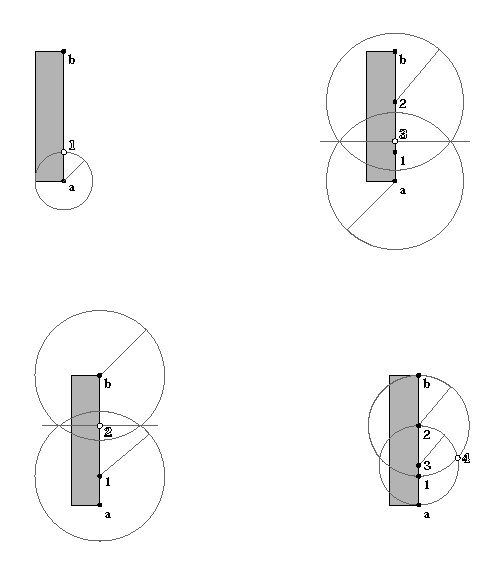The following four point construction turns the rectangle into a square of the same area.
Point 1: The intersection of line segment ab with the circle having its center at point a and radius equal to the side of the rectangle.

Point 2: The midpoint of line segment b1 ( a2 is the average of the length and width.

Point 3: The midpoint of line segment a2

Point 4: The intersection of a circle having its center at point 3 and radius equal to line segment a3, with a circle having its cetner at point 2 and radius equal to line segment b2

Line segment a4 represents the side of the square whose area equals that of the rectangle. When the width of the rectangle is the unit width, the length of a4 is the square root of line segment ab. To find the square root of a measure, start with a unit width rectangle having its length equal to that measure. Then use this construction to change the rectangle into a square. The side fo the square is the square root of the length of the rectangle.

## Geometric Proof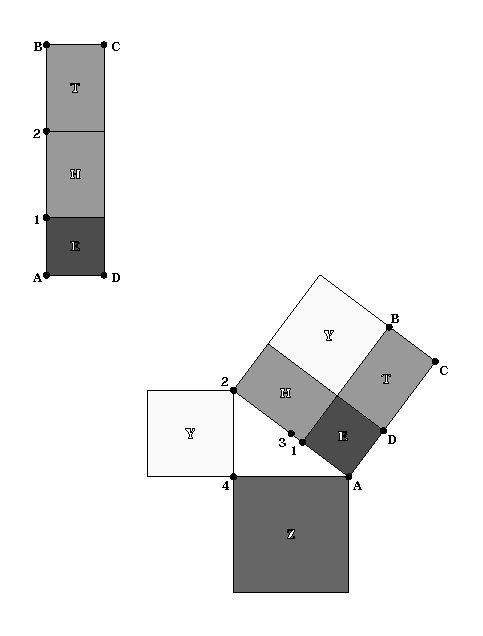Geometrically, the construction is easy to explain. Rectangle THE on the top left is equal in area to square Z on the bottom right. In the first step of the construction, rectangle THE is divided into 3 parts. Point 1 marks square E, and Point 2 divides the remainder into 2 equal rectangles: T and H. Points A, B, 1, 2, 3, and 4 correspond to the square root construction from the previous page. In the second step, the bottom picture shows rectangle THE transformed by separating rectangle T and attaching it to square E as shown. Rectangle THE is now an irregular hexagon, and also the difference of two squares. (AC and A2 are equivalent since E is a square, and H and T are equivalent rectangles.) Finally Square Z is resolved through the subtraction construction already shown.

## Compass-Only Construction of the Midpoint of a Line Segment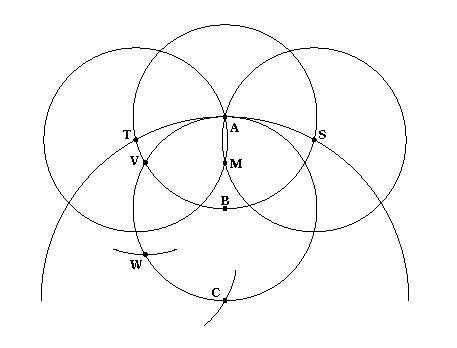The following compass-only constructions are derived from Fundamentals of Mathmatics. To determine the midpoint M of line segment AB, construct M as the inverse image of point C, through the circle of inversion (A, B).
1. Draw the two circles (A, B) and (B, A), then mark point V as the left intersection point.
2. Draw circle (V, A) and mark point W at the intersection with circle (B, A). AV = BA.
3. Draw circle (W, V) and mark point C at the intersection with circle (B, A). AC is the diameter of circle (B, A) and therefore twice the length of AB.
4. Draw circle (C, A) and mark points S and T at the intersections with circle (A, B). CA is perpendicular to ST.
5. Draw circles (S, A) and (T, A), and then mark their intersection at point M. STis perpendicular to AM therefore M lies on AC. and triange CVA is similar to triangle VMA. So AV : CA = AM : AV therefore AM = 1/2 AB.

## Compass-Only Construction of the Intersection of a Circle and a Line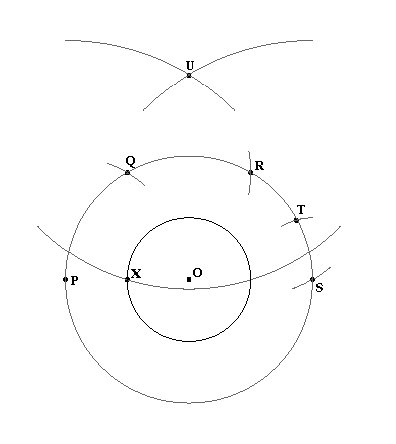To determine the points of intersection X and Y of a circle K with a line through its center O, the line being given by a point P (in addition to O). Let the radius of the circle be r, and let p = OP .

Here we are given the point P and the circle K with center O. Construct the circle through P with center O, and from P mark off the radius three times (points Q, R, S) on the circumference, and also mark off the distance ST = r. Then PT = sqare root { 4p**2 -r**2 }. Draw the circles about P and S with radius PT, thus obtaining the point U. Draw the circle with center U and radius PR = p [ square root { 3 } ] , thus obtaining the points X and Y (in all, eight circles are necessary).

## Proof of the Compass-Only Construction of the Intersection of a Circle and a Line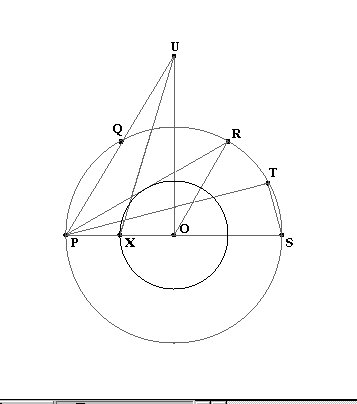Prove: X is the intersection of the circle (O, r) and the line segment OP.

Given: OP = OQ = OR = OS = PQ = QR = RS = p, UX = PR, UP = PT, OX = TS = r, angle UOP is a right angle, X lies on the circle (O, r).

## Proof

• triangle TSP is a right triangle, so line segment UP = PT = square root (4p**2 - r**2)
• angle POR is constructed as 2pi / 3 radians, because arc PR is subtended by a diameter length chord. And OR = OP = p,
so line segment PR = p {square root [ 3 ] } square root [p**2 + p**2 - 2(p)(p)cos{2pi / 3} ] = p {square root [ 3 ] }
• UX = PR = p {square root [ 3 ] }
• triangle UOP is a right triangle, so OU = square root [ 3p**2 - r**2 ]
• triangle UOX is a right triangle because OU**2 + OX** 2 = UX** 2.
• So angle OXP is a straight angle, because OX and OP are coincident. Q.E.D.

# SQUARE MULTIPLICATION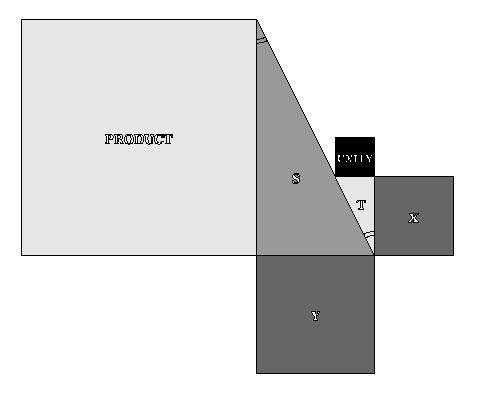Two squares X and Y may be multiplied relative to an arbitrary unity square. Let the UNITY square and X form the legs of the right triangle T. Place the square Y as shown: perpendicular and parallel to the unity square and Y. Construct another right triangle by drawing the hypotenuse from the coincident vertex of X and Y, until it intersects the extended side of X, to form triangle S. Because the hypotenuses lie on a line which traverses their parallel legs, and the alternate interior angles of two parallel lines cut by a transversal are equivalent: the triangle S is similar to T. Therefore, PRODUCT / Y = X / UNITY. And X times Y equals PRODUCT.

# SQUARE DIVISION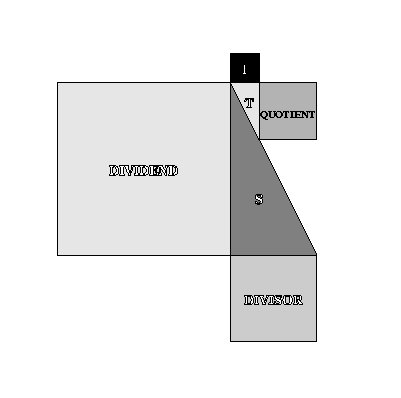The DIVIDEND square divided by the DIVISOR, relative to the UNITY square, is equal to the QUOTIENT square. This is almost identical to the multiplication construction, except the right triangle S is formed first, and the UNITY square is positioned parallel and perpendicular to the legs of triangle S. DIVIDEND / DIVISOR = QUOTIENT / UNITY. When the DIVIDEND is equivalent to the UNITY square, the QUOTIENT square is the inverse of the DIVIDEND. The multiplicative inverse is called the reciprocal. When the DIVISOR is equivalent to the UNITY square, the QUOTIENT is equivalent to the DIVIDEND. The UNITY square is the multiplicative identity element.

# SQUARE MEASURE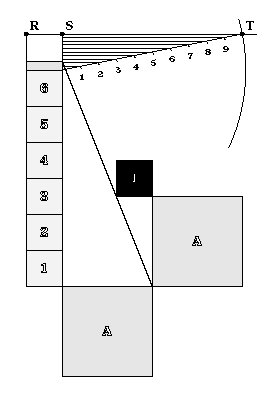The measure of square A is the number of unity squares that will fit into it. This measure isfound here by constructing a rectangle of unit width, equal in area to square A. The construction is similar to multiplying A by itself where the result would be square A squared, but it is only necessary to measure the side of the product square to obtain the measure of square A, since the measure of a side of a square is the square root of the measure of its area.

To measure a line, count off unit lengths as shown. Then proceed to obtain the next significant digit from the remainder. Construct a line ruler of ten arbitrary units, for base ten. The units may be different than the unity square. Draw a circle using the length of the ruler as radius and the center of the circle as the endpoint of the last whole unit that fit into the length of the unit rectangle. Find Point T where the circle intersects line RS. Draw lines parallel to RS from each calibration point on the ruler to obtain the next significant digit of the measure. Repeat this procedure for the next significant digit. In this case, it would be obscure to find that 6.25 is the measure of square A, because the construction would be taking place in an area which is too small to be practical.

This construction may be applied to measure the line on the side of the square that was obtained in the square root construction.

# SQUARE DISTRIBUTIVE PROPERTY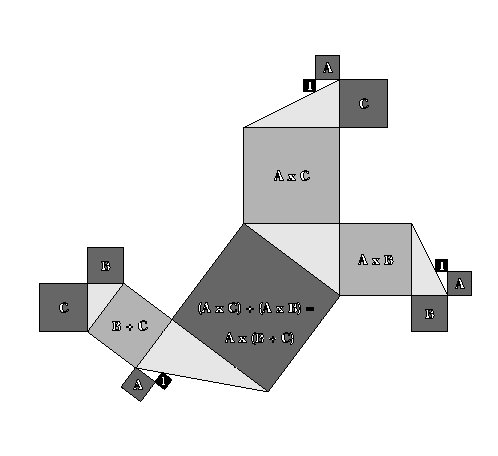The set of squares, with the operations of addition and multiplication, is 1-1 and onto the set of real numbers. Each square has exactly one real number measure, relative to an identity square. Also, by square rooting a unit rectangle, each real number has exactly one corresponding square. This correspondence is preserved under the operations of addition and multiplication by virtue of the previous constructions. Therefore the the set of squares with these operations form a complete ordered field, and the set is isomorphic to the real number set. So, squares have properties like distributive, shown below and associative, shown next.

# SQUARE MULTIPLICATIVE ASSOCIATIVE PROPERTY# CUBE ROOT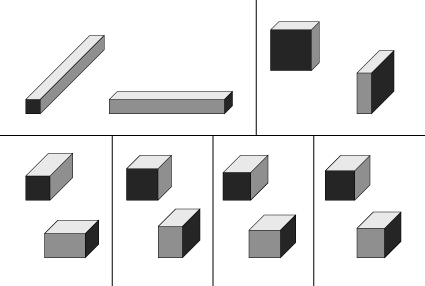The construction for the cube root works by changing a unit rectangular prism into a cube. The starting rectangular prism has unit squares on two opposite faces, while the other four are unit rectangles, having length equal to a. This construction is iterative, producing a sequence that converges to a cube with a side equal to the cube root of a.

At each stage of the sequence, transform and mold the prism by constructing a square from the unit rectangle. This transformation will retain the area, while producing, once again, a rectangular prism where two opposite faces are squares. Eventually, it approaches a cube.

The proof which is not shown uses limit theory of series and sequences to show that transformation of the rectangular prism in this manner does indeed converge to a cube. Limit theory may be used since the set of squares with the operations of multiplication and addition is isomorphic to the set of real numbers.

# SUMMARY

This paper has shown the set of squares, under the operations of addition and multiplication constructions, to be a complete ordered field. Examples of applications were shown to be a square root and cube root construction. Many orthodox mathematical concepts have been illustrated here in a simple and graphic approach. This approach can be used to teach these concepts on many levels from grade school arithmetic to college level analysis. The square root addition tessellation on page 5 illustrated the aesthetics and beauty of these concepts.

# FOOTNOTES

1. Journey Through Genius, The Great Theorems of Mathematics, William Dunham. John Wiley & Sons, NY. 1990. pp. 7.

2. Fundamentals ofMathematics, Volume 2, Geometry, Ed. Behnke, Bachmann, Fladt, and Kunle. MIT Press, Cambridge MA. 1974. Chapter 7, Geometric Constructions, W. Breidenbach and W. S<129>ss, pp. 224 - 228.

3. The Thirteen Boks of Euclid's Elements, Dover(Reprint), 1981. Proposition 14 of Book II.Return to All Raw Times Homepage